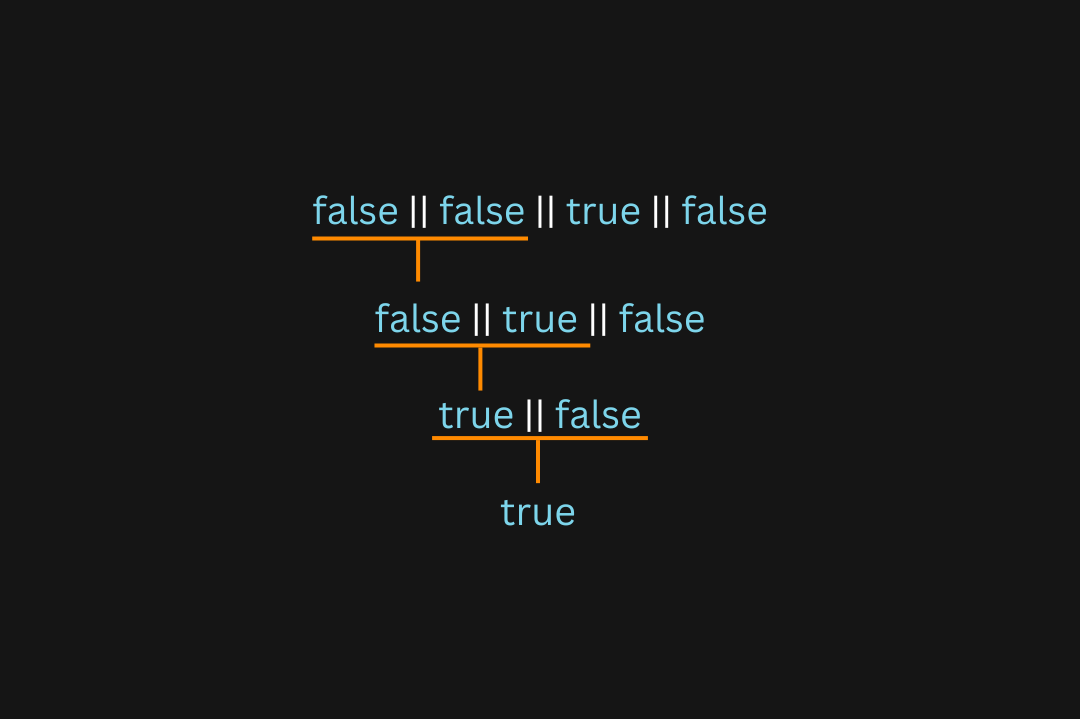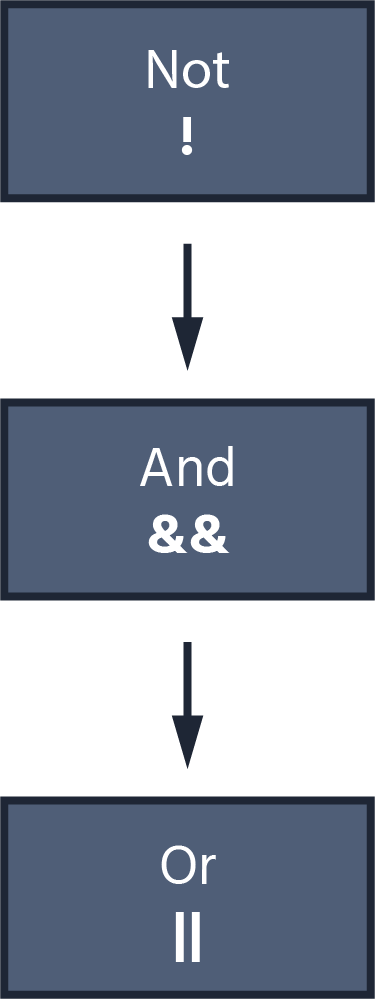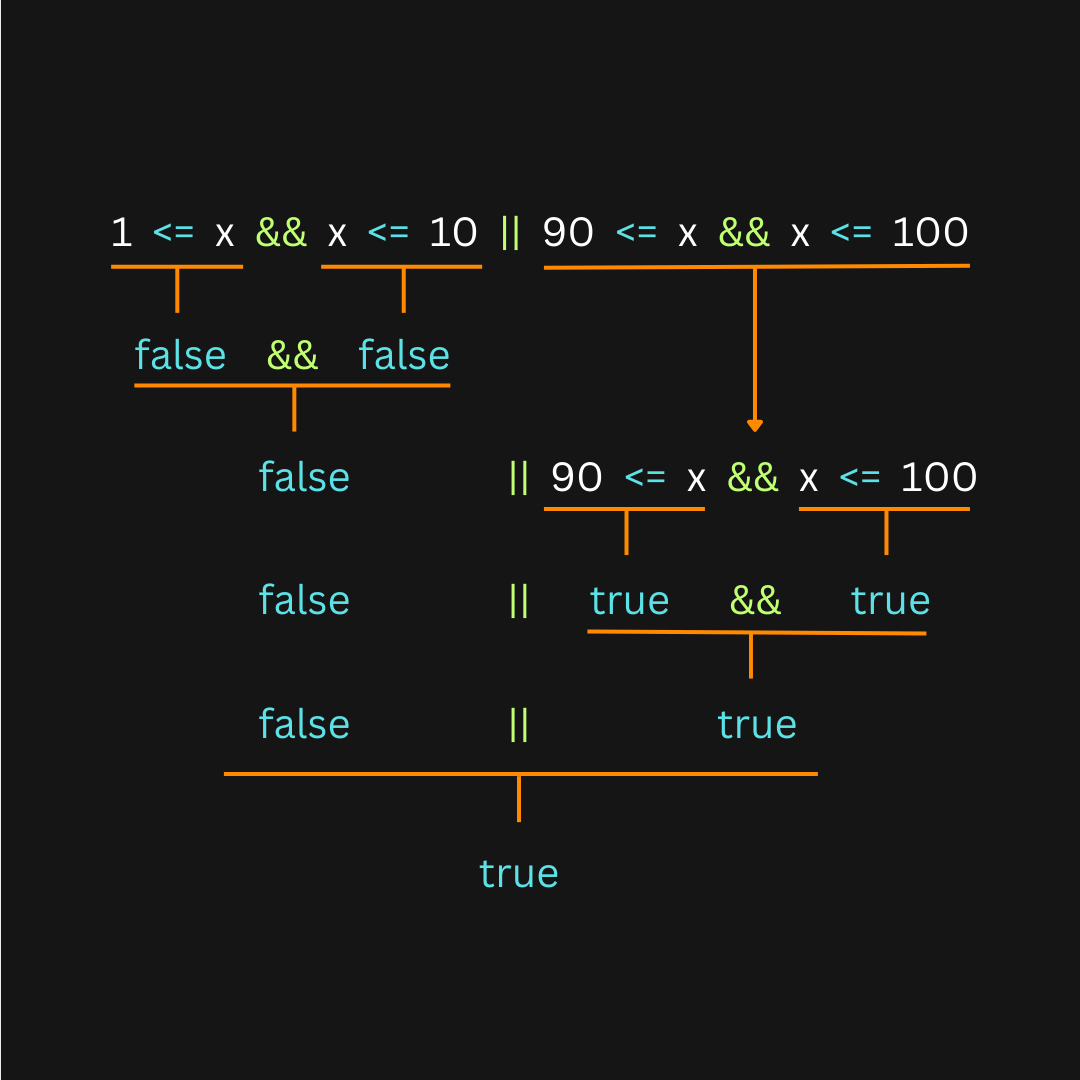Course Content

Introduction to GoLang

##Logical Operators

Another class of operators is known as logical operators. Unlike other operators that work with numerical values, logical operators are designed for handling boolean values. There are three logical operators:

 Operator Logic `!` NOT `||` OR `&&` AND

The NOT (`!`) operator is used to negate (invert) a boolean expression. Here's a simple example that includes an if-statement:index.goIn the above code, the `Print` statement won't be executed because the condition is `false` by default. However, if we include a NOT operator in the expression, it will be negated and, therefore, equivalent to `true`:index.goWe can also use the NOT (`!`) operator in more complex cases. However, we need to enclose the expressions in parentheses before negating them. For example, if we want to negate `3 > 4`, we would write `!(3 > 4)`:index.goThe OR (`||`) operator evaluates two operands and returns `true` if at least one of the operands is `true`.

Note

An operand refers to a value or expression used as input for an operator in a statement or expression. For example, in the expression `1 + 2`, the values `1` and `2` are operands. In the case of logical operators, an operand is always a boolean expression or value.

Here's an example that illustrates the basic usage of logical operators:index.goThe first statement outputs `true` because one of the operands in the expression `true || false` is `true`. In contrast, the second statement outputs `false` as both operands are `false` in that expression. Below is the truth table for the logical OR operation:

Note

A truth table is a logical representation that displays all possible combinations of inputs and their corresponding output values.

 Input Output `true || true` true `true || false` true `false || true` true `false || false` false

We can chain logical OR operators to combine multiple operands. In this case, the expression is evaluated from left to right:index.goThe order of evaluation for the above expression is as follows:Note

By default, all logical expressions are evaluated from left to right.

The logical AND (`&&`) operator is similar to the OR (`||`) operator, but it returns `true` only if both operands have a value of `true`. The truth table for the logical AND (`&&`) operation is provided below:

 Input Output `true && true` true `true && false` false `false && true` false `false && false` false

Here's a more complex example that demonstrates a practical use of logical operators. Take a moment to examine the code and understand how it works:index.goPlease note that in the above example, we use multiple operators and values to create a more complex condition. During execution, the expression is evaluated based on the operator's priority. High-priority operators are evaluated first, and it's important to understand the order in which they are processed. Comparison operators have higher priority than logical operators.

Below is a diagram illustrating the priority ranking of logical operators:The following diagram illustrates how the expression `1 <= x && x <= 10 || 90 <= x && x <= 100` is evaluated:#What of the following expressions can we use in the if-statement to check if a shape is a square based on its `width` and `height` ?

## Select a few correct answers

Everything was clear?

Section 3. Chapter 4